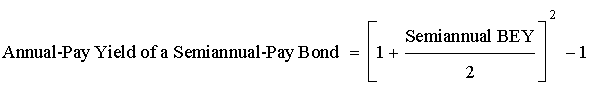### Seeing is believing!

Before you order, simply sign up for a free user account and in seconds you'll be experiencing the best in CFA exam preparation.

### Subject 5. Bond Equivalent Yield

Periodic bond yields for both straight and zero-coupon bonds are conventionally computed based on semi-annual periods, as U.S. bonds typically make two coupon payments per year. For example, a zero-coupon bond with a maturity of five years will mature in 10 6-month periods. The periodic yield for that bond, r, is indicated by the equation Price = Maturity value x (1 + r)-10. This yield is an internal rate of return with semi-annual compounding. How do we annualize it?

The convention is to double it and call the result the bond's yield to maturity. This method ignores the effect of compounding semi-annual YTM, and the YTM calculated in this way is called a bond-equivalent yield (BEY).

However, yields of a semi-annual-pay and an annual-pay bond cannot be compared directly without conversion. This conversion can be done in one of the two ways:

• Convert the bond-equivalent yield of a semi-annual-pay bond to an annual-pay bond.• Convert the equivalent annual yield of an annual-pay bond to a bond-equivalent yield.Example

• A Eurobond pays coupon annually. It has an annual-pay YTM of 8%.
• A U.S. corporate bond pays coupon semi-annually. It has a bond equivalent YTM of 7.8%.
• Which bond is more attractive, if all other factors are equal?

Solution 1

• Convert the U.S. corporate bond's bond equivalent yield to an annual-pay yield:
• Annual-pay yield = [1 + 0.078/2]2 - 1 = 7.95% < 8%
• The Eurobond is more attractive since it offers a higher annual-pay yield.

Solution 2

• Convert the Eurobond's annual-pay yield to a bond equivalent yield (BEY):
• BEY = 2 x [(1 + 0.08)0.5 - 1] = 7.85% > 7.8%
• The Eurobond is more attractive since it offers a higher bond equivalent yield.

#### Practice Question 1

Which of the following is incorrect?

A. BEY is the annual yield calculated from semi-annual, quarterly, or monthly discount-bond or note yields.
B. Bond Equivalent Yield for a semi-annual pay bond is calculated as twice the six month yield.
C. Bond Equivalent Yield for a semi-annual pay bond is more than the real annual return from holding the bond till maturity (assuming no default).

BEY will be less than the real return as (1 + x)2 is greater than 1 + 2x.

#### Practice Question 2

The bond equivalent yield for a semi-annual pay bond is most likely ______.

A. equal to the effective annual yield
B. more than the effective annual yield
C. equal to double the semi-annual yield to maturity

The bond equivalent yield for a semi-annual pay bond is equal to double the semi-annual yield to maturity.

#### Practice Question 3

What is the bond equivalent yield of a 7% annual-pay bond with semi-annual compounding?

A. 6.882%
B. 7.000%
C. 7.123%

2[(1.07)0.5 -1] = 6.8826%

#### Practice Question 4

Why is the bond-equivalent yield of an annual-pay bond always less than the annual-pay rate?

A. The annual-pay bond is divided by two for the semi-annual equivalent.
B. The annual-pay bond will pay twice as much interest in one payment.
C. The bond equivalent yield compounds only half as often for the annual-pay bond.
D. The square root of the annual-pay yield is taken to convert from one to two pay periods in a year and maintain the same yield when the two periods are compounded back to an annual basis.

#### Practice Question 5

A bond equivalent yield is a money market rate stated on a ______ rate basis.

A. 360-day discount

#### Practice Question 6

The bond equivalent yield of a 120-day banker's acceptance quoted at a discount rate of 4.0% for a 360 day-year is closest to ______.

A. 3.89%
B. 3.96%
C. 4.10%